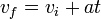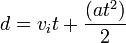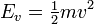# Velocity

Velocity (symbol: v) is a vector measurement of the rate and direction of motion. The scalar absolute value (magnitude) of velocity is speed. Velocity can also be defined as rate of change of displacement or just as the rate of displacement, depending on how the term displacement is used. It is thus a vector quantity with dimension length/time. In SI units this is metre per second.

In mechanics the average speed v of an object moving a distance d during a time interval t is described by the simple formula:$v = \frac{d}{t} \,$

The instantaneous velocity vector v of an object whose position at time t is given by x(t) can be computed as the derivative$\mathbf{v} = \frac {d\mathbf{x}}{dt} \,$

Acceleration is the rate of change of an object's velocity over time. The average acceleration of a of an object whose speed changes from vi to vf during a time interval t is given by:$a = \frac {( v_f - v_i )} {t} \,$

The instantaneous acceleration vector a of an object whose position at time t is given by x(t) is$\mathbf{a} = \frac {d\mathbf{v}} {dt} = \frac {d^2\mathbf{x}} {d t^2} \,$

The final velocity vf of an object which starts with velocity vi and then accelerates at constant acceleration a for a period of time t is:$v_f = v_i + a t \,$

The average velocity of an object undergoing constant acceleration is (vi + vf)/2. To find the displacement d of such an accelerating object during a time interval t, substitute this expression into the first formula to get:$d = t \times \frac { ( v_i + v_f )} {2} \,$

When only the object's initial velocity is known, the expression$d = v_i t + \frac {( a t^2 )} {2} \,$

can be used. These basic equations for final velocity and displacement can be combined to form an equation that is independent of time:$v_f^2 = v_i^2 + 2 a d \,$

The above equations are valid for both classical mechanics and special relativity. Where classical mechanics and special relativity differ is in how different observers would describe the same situation. In particular, in classical mechanics, all observers agree on the value of t and the transformation rules for position create a situation in which all non-accelerating observers would describe the acceleration of an object with the same values. Neither is true for special relativity.

The kinetic energy (movement energy) of a moving object is linear with both its mass and the square of its velocity:$E_{v} = \begin{matrix} \frac{1}{2} \end{matrix} mv^2$

The kinetic energy is a scalar quantity.

## Polar coordinates

In connection with polar coordinates, two dimensional velocity can be decomposed into radial velocity, away from or toward the origin, and transverse velocity, in the perpendicular direction, changing the direction of the body from the origin, and equal to the distance to the origin times the angular velocity.

Angular momentum in scalar form is the distance to the origin times the transverse speed, or equivalently, the distance squared times the angular speed, with a plus or minus to distinguish clockwise and anti-clockwise direction.

If forces are in radial direction only, as in the case of a gravitational orbit, angular momentum is constant, hence transverse speed is inversely proportial to the distance, angular speed is inversely proportial to the distance squared, and the rate at which area is swept out is constant (Kepler's second law).